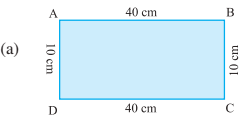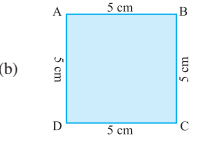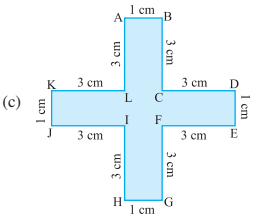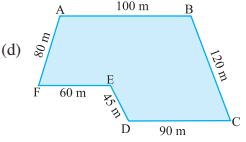# Find the perimeter of the following figures:Perimeter = AB + BC + CD + DA          = __+__+__+__          = ______Perimeter = AB + BC + CD + DA         = __ + __ + __+ __         = ______Perimeter = AB + BC + CD + DE + EF + FG + GH +HI + IJ + JK + KL + LA           = __ + __ +__ + __ + __ +  __ + __ + __ +__+ __ + __ + __           = ______Perimeter = AB + BC + CD + DE + EF+ FA            = __ + __ + __ + __ + __ + __            = ______

Find the perimeter of the following figures:Perimeter = AB + BC + CD + DA
= 40+10+40+10
= 100Perimeter = AB + BC + CD + DA
=5+5+5+5=20Perimeter = AB + BC + CD + DE + EF + FG + GH +HI + IJ + JK + KL + LA
=1+3+3+1+3+3+1+3+3+1+3+3=28 cmPerimeter = AB + BC + CD + DE + EF+ FA
=100 + 120 + 90 + 45+ 60 +80=495 cm

## Related Chapters

### Preparation Products

##### JEE Main Rank Booster 2021

This course will help student to be better prepared and study in the right direction for JEE Main..

₹ 13999/- ₹ 9999/-
##### Rank Booster NEET 2021

This course will help student to be better prepared and study in the right direction for NEET..

₹ 13999/- ₹ 9999/-
##### Knockout JEE Main April 2021 (Easy Installments)

An exhaustive E-learning program for the complete preparation of JEE Main..

₹ 4999/-
##### Knockout NEET May 2021

An exhaustive E-learning program for the complete preparation of NEET..

₹ 22999/- ₹ 14999/-